Updating search results...

# 30 Results

View
Selected filters:
• triangle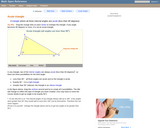Rating
0.0 stars

An interactive applet and associated web page that demonstrate the three types of triangle: acute, obtuse and right. The applet shows a triangle that is initially acute (all angles less then 90 degrees) which the user can reshape by dragging any vertex. There is a message changes in real time while the triangle is being dragged that tells if the triangle is an acute, right or obtuse triangle and gives the reason why. By experimenting with the triangle student can develop an intuitive sense of the difference between these three classes of triangle. Applet can be enlarged to full screen size for use with a classroom projector. This resource is a component of the Math Open Reference Interactive Geometry textbook project at http://www.mathopenref.com.

Subject:
Geometry
Mathematics
Material Type:
Simulation
Provider:
Math Open Reference
Author:
John Page
05/07/2019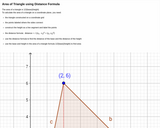Unrestricted Use
CC BY
Rating
0.0 stars

Definition of area of triangle, image of triangle on coordinate grid with height constructed. Area of triangle calculated using distance formula.

Subject:
Geometry
Mathematics
Material Type:
Interactive
Provider:
Michigan Virtual
07/19/2019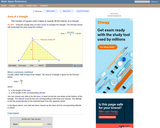Rating
0.0 stars

An interactive applet and associated web page that explain the area of a triangle. The applet shows a triangle that can be reshaped by dragging any vertex. As it changes, the area is continually recalculated using the 'half base times height' method. The triangle has a fixed square grid in its interior that can be used to visually estimate the area for later correlation with the calculated value. The calculation can be hidden while estimation is in progress. The text page has links to a similar page that uses Heron's Formula to compute the area. Applet can be enlarged to full screen size for use with a classroom projector. This resource is a component of the Math Open Reference Interactive Geometry textbook project at http://www.mathopenref.com.

Subject:
Geometry
Mathematics
Material Type:
Simulation
Provider:
Math Open Reference
Author:
John Page
05/07/2019Rating
0.0 stars

This student interactive, from Illuminations, allows students to explore the conditions that guarantee uniqueness of a triangle, quadrilateral, or pentagon regardless of location or orientation. Each set of conditions results in a new congruence theorem.

Subject:
Math 2
Mathematics
Material Type:
Activity/Lab
Interactive
Provider:
National Council of Teachers of Mathematics
Author:
Illuminations
02/26/2019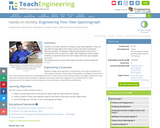Educational Use
Rating
0.0 stars

Students use simple materials to design an open spectrograph so they can calculate the angle light is bent when it passes through a holographic diffraction grating. A holographic diffraction grating acts like a prism, showing the visual components of light. After finding the desired angles, students use what they have learned to design their own spectrograph enclosure.

Subject:
Applied Science
Engineering
Material Type:
Activity/Lab
Provider:
TeachEngineering
Provider Set:
TeachEngineering
10/14/2015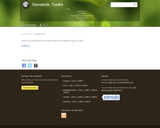Rating
0.0 stars

In this assessment task students are given a geoboard and rubberband and are asked to make a square, a triangle and a hexagon. Students can be assessed in a small group or whole class. A checklist and rubric are provided.

Subject:
Mathematics
Material Type:
Activity/Lab
Provider:
Hawaii State Department of Education
Author:
Hawaii State Department of Education
02/26/2019Rating
0.0 stars

This resource describes activities using interactive geoboards to help students identify simple geometric shapes, describe their properties, and develop spatial sense. The first part, Making Triangles, focuses attention on the concept of triangle, helping students understand the mathematical meaning of a triangle and the idea of congruence, or sameness, in geometry. In the next part, Creating Polygons, students make and compare a variety of polygons, describing the salient properties of the shapes they create.

Subject:
Mathematics
Material Type:
Activity/Lab
Interactive
Provider:
National Council of Teachers of Mathematics
Author:
National Council of Teachers of Mathematics
02/26/2019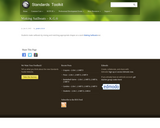Rating
0.0 stars

In this assessment task students are asked to use pattern blocks to make sailboats that are on a workmat. They are asked to show different ways to fill the same shape for example a student may use one trapezoid and three triangles to create the shape. The teacher will be able to observe whether a student uses flips, slides or turns purposefully. The workmat and rubric are provided.

Subject:
Mathematics
Material Type:
Activity/Lab
Provider:
Hawaii State Department of Education
Author:
Hawaii State Department of Education
02/26/2019Only Sharing Permitted
CC BY-NC-ND
Rating
0.0 stars

This resource is from Tools 4 NC Teachers. This document is the About the Cluster document for Cluster 5 created by the authors of the NC2ML Instructional Frameworks. This document should be read prior to teaching Cluster 5.

Subject:
Mathematics
Material Type:
Reference Material
07/28/2020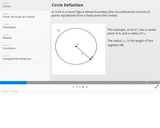Unrestricted Use
CC BY
Rating
0.0 stars

Proof that the sum of angles of a triangle equals 180 degrees using vertical angles and corresponding angles.

Subject:
Geometry
Mathematics
Material Type:
Interactive
Provider:
Michigan Virtual
07/19/2019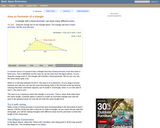Rating
0.0 stars

An interactive applet and associated web page that show the relationship between the perimeter and area of a triangle. It shows that a triangle with a constant perimeter does NOT have a constant area. The applet has a triangle with one vertex draggable and a constant perimeter. As you drag the vertex, it is clear that the area varies, even though the perimeter is constant. Optionally, you can see the path traced by the dragged vertex and see that it forms an ellipse. A link takes you to a page where this effect is exploited to construct an ellipse with string and pins. The applet can be enlarged to full screen size for use with a classroom projector. This resource is a component of the Math Open Reference Interactive Geometry textbook project at http://www.mathopenref.com.

Subject:
Geometry
Mathematics
Material Type:
Simulation
Provider:
Math Open Reference
Author:
John Page
05/07/2019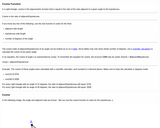Unrestricted Use
CC BY
Rating
0.0 stars

Description of cosine function with image, calculation of length of hypotenuse with known angle and adjacent side, calculation of angle with known adjacent and hypotenuse sides. Explanation of inverse cosine. Interactive fill in the blank of main points.

Subject:
Geometry
Mathematics
Material Type:
Interactive
Provider:
Michigan Virtual
07/19/2019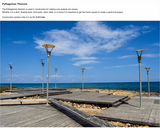Unrestricted Use
CC BY
Rating
0.0 stars

Image of real life project using Pythagorean Theorem. Definition of theorem, directions with images on how to use theorem to square a construction project.

Subject:
Geometry
Mathematics
Material Type:
Interactive
Provider:
Michigan Virtual
07/19/2019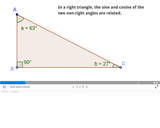Unrestricted Use
CC BY
Rating
0.0 stars

Course presentation showing relationship of sine and cosine of angles in a right triangle

Subject:
Geometry
Mathematics
Material Type:
Interactive
Provider:
Michigan Virtual
07/19/2019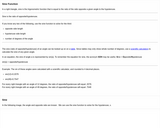Unrestricted Use
CC BY
Rating
0.0 stars

Description of sine function with image, calculation of length of hypotenuse with known angle and opposite side, calculation of angle with known opposite and hypotenuse sides. Explanation of inverse sine. Interactive fill in the blank of main points.

Subject:
Geometry
Mathematics
Material Type:
Interactive
Provider:
Michigan Virtual
07/19/2019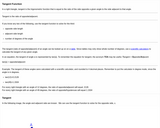Unrestricted Use
CC BY
Rating
0.0 stars

Description of tangent function with image, calculation of length of opposite side with known angle and adjacent side, calculation of angle with known opposite and adjacent sides. Explanation of inverse tangent Interactive fill in the blank of main points.

Subject:
Geometry
Mathematics
Material Type:
Interactive
Provider:
Michigan Virtual
07/19/2019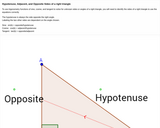Unrestricted Use
CC BY
Rating
0.0 stars

Trigonometry ratios of right triangle, image of right triangle with hypotenuse, opposite, and adjacent sides labeled with hotspot information. Interactive Drag and drop image of labeling sides of right triangle

Subject:
Geometry
Mathematics
Material Type:
Interactive
Provider:
Michigan Virtual
07/19/2019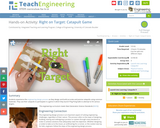Educational Use
Rating
0.0 stars

Students experience the engineering design process as they design and build accurate and precise catapults using common materials. They use their catapults to participate in a game in which they launch Ping-Pong balls to attempt to hit various targets.

Subject:
Applied Science
Engineering
Material Type:
Activity/Lab
Provider:
TeachEngineering
Provider Set:
TeachEngineering
Author:
Carleigh Samson
Jake Crosby
Jonathan McNeil
Malinda Schaefer Zarske
William Surles
09/18/2014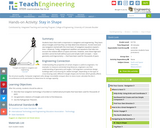Educational Use
Rating
0.0 stars

Students learn that math is important in navigation and engineering. They learn about triangles and how they can help determine distances. Ancient land and sea navigators started with the most basic of navigation equations (speed x time = distance). Today, navigational satellites use equations that take into account the relative effects of space and time. However, even these high-tech wonders cannot be built without pure and simple math concepts â basic geometry and trigonometry â that have been used for thousands of years.

Subject:
Applied Science
Engineering
Material Type:
Activity/Lab
Provider:
TeachEngineering
Provider Set:
TeachEngineering
Author:
Janet Yowell
Jeff White
Malinda Schaefer Zarske
Matt Lippis
10/14/2015Conditional Remix & Share Permitted
CC BY-NC-SA
Rating
0.0 stars

This resource is part of Tools4NCTeachers.

This list contains links to parent videos, student videos, games, books, and activities aligned to Kindergarten, Cluster 1.

Subject:
Mathematics
Material Type:
Activity/Lab
Game